Courses

# Test: Previous Year Questions: Structure Of Atom

## 28 Questions MCQ Test Chemistry for JEE | Test: Previous Year Questions: Structure Of Atom

Description
This mock test of Test: Previous Year Questions: Structure Of Atom for JEE helps you for every JEE entrance exam. This contains 28 Multiple Choice Questions for JEE Test: Previous Year Questions: Structure Of Atom (mcq) to study with solutions a complete question bank. The solved questions answers in this Test: Previous Year Questions: Structure Of Atom quiz give you a good mix of easy questions and tough questions. JEE students definitely take this Test: Previous Year Questions: Structure Of Atom exercise for a better result in the exam. You can find other Test: Previous Year Questions: Structure Of Atom extra questions, long questions & short questions for JEE on EduRev as well by searching above.
QUESTION: 1

Solution:

∆x*∆mv=h/4π

QUESTION: 2

### Energy of H–atom in the ground state is -13.6 eV , Hence energy in the second excited state is–

Solution:

The formula to calculate the energy is minus 13.6 multiply by z2 divided by n2.Here in second excited state n is equal to 3 and z for hydrogen is 1. So -13.6/9 = -1.51 ev

QUESTION: 3

### Uncertainty in position of a particle of 25 g in space is 10-5 m. Hence uncertainty in velocity (ms-1) is (Planck's constant h = 6.6 × 10-34 Js)                                                            [AIEEE- 2002]

Solution:

∆p×∆x = h/4π
Or ∆v = h/4π∆xm      (since p = mv)
=6.6×10-34Js/4×3.14×25×10-3kg ×10-5

QUESTION: 4

The orbital angular momentum for an electron revolving in an orbit is given by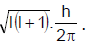. This momentus for an s-electron will be given by -                                                                                 [AIEEE- 2003]

Solution:

The value of l (azimuthal quantum number) for s electron is equal to zero.QUESTION: 5

In Bohr series of lines of hydrogen spectrum, third line from the red end corresponds to where one of the following inter-orbit jumps of electron for Bohr orbits in an atom of hydrogen.                [AIEEE- 2003]

Solution:

The electron has minimum energy in the first orbit and its energy increases as n increases. Here n represents number of orbit, i.e. 1st, 2nd, 3rd .... The third line from the red end corresponds to yellow region i.e. 5. In order to obtain less energy electron tends to come in 1st or 2nd orbit. So jump may be involved either 5 → 1 or 5 → 2.

QUESTION: 6

The de Broglie wavelength of a tennis ball mass 60 g moving with a velocity of 10 mt. per second is approximately -                                                                                             [AIEEE- 2003]

Solution: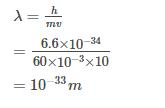*Multiple options can be correct
QUESTION: 7

Which of the following sets of quantum numbers is correct for an electron in 4f orbital ?          [AIEEE- 2004]

Solution:

For 4f orbital electrons, n = 4

s p d f

l = 3 (because 0 1 2 3 )

m = +3, +2, +1, 0, –1, –2, –3

s = ±1/2.

QUESTION: 8

Consider the ground state of Cr atom (Z = 24). The numbers of electrons with the azimuthal quantum numbers, l = 1 and 2 are, respectively                                                                  [AIEEE- 2004]

Solution:QUESTION: 9

The wavelength of the radiation emitted, when in a hydrogen atom electron falls from infinity to stationary state 1, would be (Rydberg constant = 1.097×107 m-1)                                           [AIEEE- 2004]

Solution: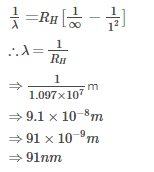QUESTION: 10

Which one of the following sets of ions represents the collection of isoelectronic species ? [AIEEE- 2004]

Solution:

Isoelectronic species are those which have same number of electrons.
K= 19 – 1 = 18 ; Ca2+ = 20 – 2 = 18
Sc3+ = 21 – 3 = 18 ; Cl– = 17 + 1 = 18
Thus all these ions have 18 electrons in them.

QUESTION: 11

In a multi-electron atom, which of the following orbitals described by the three quantum members will have the same energy in the absence of magnetic and electric fields ?                [AIEEE- 2005]

(a) n = 1, l = 0, m = 0

(b) n = 2, l = 0, m = 0

(c) n = 2, l = 1, m = 1

(d) n = 3, l = 2, m = 1

(e) n = 3, l = 2, m = 0

Solution:

Orbitals having (n+l) same possess same energy in absence of magnetic field.

Hence (c) is the correct answer.

QUESTION: 12

Of the following sets which one does NOT contain isoelectronic species ?               [AIEEE- 2005]

Solution:

In the first example (CN-, N2, C22-) we have 14 electrons. In second (PO43-, SO42-, ClO4-) we have 50 electrons. In the third (BO33-, CO32-,NO3-), we have 32 electrons. While n last case, although we have 32 electrons in CO32- and NO3- but SO32- has 42 electrons. So, the last case has no isoelectronic species.

QUESTION: 13

According to Bohr's theory, the angular momentum of an electron in 5th orbit is -        [AIEEE 2006]

Solution:

The angular momentum (mvr) of electron in nth orbit is equal to nh/2π
For the electron in 5th orbit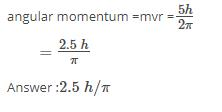QUESTION: 14

Uncertainty in the position of an electron (mass = 9.1 × 10-31 kg) moving with a velocity 300 m/s, accurate upto 0.001 %, will be (h = 6.63 × 10-34 Js)                                         [AIEEE 2006]

Solution:QUESTION: 15

Which of the following sets of quantum numbers represents the highest energy of an atom ? [AIEEE 2007]

Solution: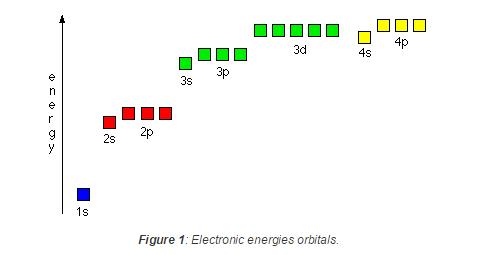The aufbau principle explains how electrons fill low energy orbitals (closer to the nucleus) before they fill higher energy ones. Where there is a choice between orbitals of equal energy, they fill the orbitals singly as far as possible(Hunds rule). The diagram summarizes the energies of the orbitals up to the 4p level.Therefore 3d orbitals have higher energy than the other orbitals in the options.

So answer is: (2) n = 3, l = 2, m = 1, s = +1⁄2

QUESTION: 16

The ionization enthalpy of hydrogen atom is 1.312 × 106 J mol-1. The energy required to excite the electron in the atom from n = 1 to n = 2 is                                                       [AIEEE 2008]

Solution: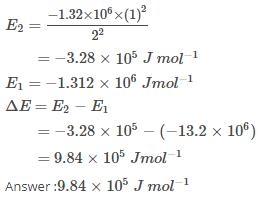QUESTION: 17

In an atom, an electron is moving with a speed of 600 m/s with an accuracy of 0.005%. Certainity with which the position of the electron can be located is (h = 6.6×10-34 kg m2s-1 ,mass of electron, em = 9.1×10-31 kg )    [AIEEE 2009]

Solution: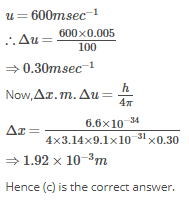QUESTION: 18

Calculate the wavelength (in nanometer) associated with a proton moving at 1.0×103ms-1

(Mass of proton = 1.67×10-27 kg and h = 6.63×10-34 Js ) :                                        [AIEEE 2009]

Solution: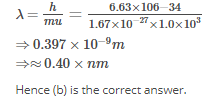QUESTION: 19

The energy required to break one mole of Cl - Cl bonds in Cl2 is 242 kJ mol-1. The longest wavelength of light capable of breaking a single Cl - Cl bond is(c = 3 x 108 ms-1 and NA = 6.02 x 1023 mol-1)                                                                                                                                                                       [AIEEE 2010]

Solution: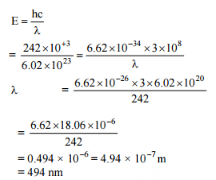QUESTION: 20

Ionisation energy of He+ is 19.6 x 10-18 J atom-1. The energy of the first stationary state (n = 1) of Li2+ is

[AIEEE 2010]

Solution: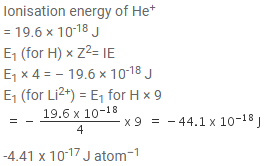QUESTION: 21

Iron exhibits +2 and +3 oxidation states. Which of the following statements about iron is incorrect ?

[AIEEE 2012]

Solution:

Ferrous oxide is more basic in nature than ferric oxide. Ferrous compounds are relatively more ionic than the corresponding ferric compounds. Ferrous compounds are less volatile than the corresponding ferric compounds as ferrous compound is more ionic.

But ferric compounds are more easily hydrolyzed than the corresponding ferrous compounds due to their more positive charge.

QUESTION: 22

The electrons identified by quantum numbers 'n' and l :                                  [AIEEE 2012]

(a) n = 4, l = 1

(b) n = 4, l = 0

(c) n = 3, l = 2

(d) n = 3, l = 1

can be placed in order of increasing energy as :

Solution:

a.) (n + l) = 4 + 1 = 5
b.) (n + l) = 4 + 0 = 4
c.) (n + 1) = 3 + 2 = 5
d.) (n + 1) = 3 + 1 = 4

Higher the value of (n+1), higher the energy. if (n+1) are same, sub orbit with a lower value of n has lower energy. Thus,

3p<4s<3d<4p

QUESTION: 23

The standard reduction potentials for Zn2+/Zn, Ni2+/Ni, and Fe2+/Fe are -0.76, -0.23 and -0.44 V respectively. The reaction X + Y2+ → X2+ + Y will be spontaneous when:             [AIEEE 2012]

Solution:

X = Zn, Y = Ni
Zn + Ni2+  →Zn2+ + Ni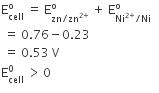QUESTION: 24

The first ionisation potential of Na is 5.1 eV. The value of electron gain enthalpy of Na+ will be:

[AIEEE 2013]

Solution:

Na → Na+ + e- (first IE)
Na+ + e- →Na
Since reaction is reverse, so electron gain enthalpy of Na+
ΔH (eg)=-5.1 eV

QUESTION: 25

Energy of an electron is given by E = -2.178 × 10-18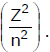Wavelength of light required to excite an electron in an hydrogen atom from level n = 1 to n = 2 will be (h = 6.62 × 10-34 Js and c = 3.0 × 108 ms-1)

[AIEEE 2013]

Solution: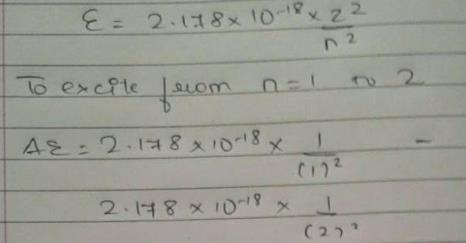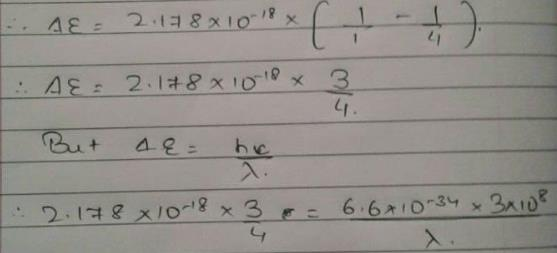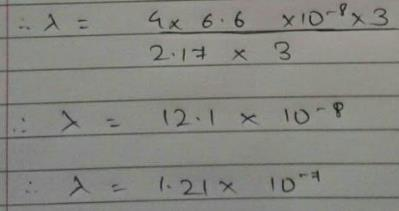QUESTION: 26

Which of the following is the wrong statement ?                                                                       [AIEEE 2013]

Solution:

'In solid state, ozone is violet-black.' Ozone does not exist in solid state, thus this is the incorrect statement.

QUESTION: 27

Which of the following arrangements does not represent the correct order of the property stated against it?

[AIEEE 2013]

Solution:

B) V2+<Cr2+<Mn​2+<Fe2+ : paramagnetic behaviour
Electronic configuration of V2+ = 3d3   ; n=3
Electronic configuration of Cr2+=3d4    ; n=4
Electronic configuration of Mn2+=3d5   ; n=5
Electronic configuration of Fe2+=3d6   ;  n=4
Here, Mn2+ has maximum number of unpaired electrons and so its most paramagentic. Therefore the correct order is :
V2+<Cr2+<Fe2+<Mn​2+

C) Ni2+<Co2+<Fe2+<Mn2+ : Ionic size
We know for the same oxidation state, the ionic radii generally decrease with increase in nuclear charge and the nuclear charge increase as we move across a period. Therefore, the given order is correct.

D) Co3+<Fe3+<Cr3+<Sc3+ : Stability in aqueous solution
The lower the electrode potential, i.e. more negative the standard reduction potential of the electrode, the more stable is the transition metal in aqueous solution. The value becomes less negative across the series. Therefore, the given order is correct.

A)Sc<Ti<Cr<Mn : number of oxidation states
The given order is correct as Mn shows maximum number of oxidation states i.e. upto +7 (due to its configuration 3d5 4s2 it can have maximum oxidation states). Sc on the other hand shows least oxidation state.

Therefore, (B) doesnot show correct order of property.

QUESTION: 28

In a hydrogen like atom electron make transition from an energy level with quantum number n to another with quantum number (n - 1). If n >> 1, the frequency of radiation emitted it proportional to   [AIEEE 2013]

Solution:

The energy transition of hydrogen atom is given by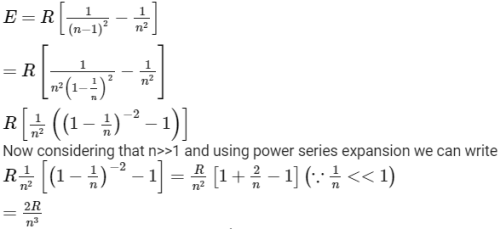So energy will be proportional to 1/n3, hence B is correct answer.